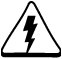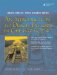# Returning References from Functions

Sometimes it can be very useful to design a function so that it returns a reference. For example, when we overload the insertion operator, operator<<(ostream&, NewType), we always return a reference to the output stream. This makes it possible to chain operations like this:

```cout << thing1 << thing2 << thing3 ... ;
```

A reference return (especially of *this) is used to provide lvalue behavior for member functions.

As with reference parameters, it is possible to protect a reference return by specifying that the object it aliases is const.

Example 5.15 captures the essence of reference returns.

Example 5.15. src/reference/maxi.cpp

 ```#include using namespace std; int& maxi(int& x, int& y) { return (x > y) ? x : y; } int main() { int a = 10, b = 20; maxi(a,b) = 5; <-- 1 maxi(a,b) += 6; <-- 2 ++maxi(a, b) ; <-- 3 cout << a << ' ' << b << endl; return 0; } Output: 17 5 ```   (1)Assigns the value 5 to b. (2)Increases a by 6. a is now 16. (3)Increments a by 1.

As we see in the main() function, the reference return value of the function maxi() makes the expression maxi(a,b) into a modifiable lvalue.Be very careful that your function does not return a reference to a temporary (local) object. A moment's thought should make that restriction clear: When the function returns, all of its local variables are destroyed.

```int& max(int i,int j) {
int retval = i > j ? i : j;

return retval;
}
```

Code like the above may generate a compiler warning (if you are lucky). Alas, the compiler does not consider it an error.

```badmax.cpp:4: warning: reference to local variable 'retval' returned
```

A more practical example showing the benefits of reference returns is coming up in Example 5.16, which defines some common operators for vectors.